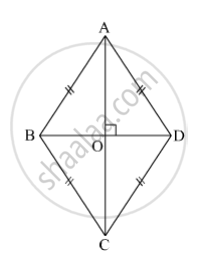SSC (English Medium) Class 8Maharashtra State Board
Share

# Lengths of Diagonals of a Rhombus Abcd Are 16 Cm and 12 Cm. Find the Side and Perimeter of the Rhombus. - SSC (English Medium) Class 8 - Mathematics

ConceptSome Special Parallelograms Concept of Rhombus

#### Question

Lengths of diagonals of a rhombus ABCD are 16 cm and 12 cm. Find the side and perimeter of the rhombus.

#### Solution

ABCD is a rhombus.Here, segment AC and segment BD are the diagonals of the rhombus ABCD.

l(AC) = 16 cm and l(BD) = 12 cm.

Diagonals of a rhombus are perpendicular bisectors of each other.

∴ m∠AOD = 90º

Also, l(OA) = $\frac{1}{2}$l(AC) = $\frac{1}{2}$× 16 = 8 cm

l(OD) = $\frac{1}{2}$l(BD) =  1/2× 12 = 6 cm

In right ∆AOD,

l(AD)2 = l(OA)+ l(OD)2               (Pythagoras theorem)

⇒ l(AD)= 64 + 36 = 100

⇒ l(AD) = $\sqrt{100}$= 10 cm

All sides of a rhombus are equal.

∴ Perimeter of the rhombus ABCD = 4 × Side of a rhombus = 4 × 10 = 40 cm

Thus, the side and perimeter of the rhombus are 10 cm and 40 cm, respectively.

Is there an error in this question or solution?

#### APPEARS IN

Balbharati Solution for Balbharati Class 8 Mathematics (2019 to Current)
Chapter 8: Quadrilateral : Constructions and Types
Practice Set 8.2 | Q: 5 | Page no. 47
Solution Lengths of Diagonals of a Rhombus Abcd Are 16 Cm and 12 Cm. Find the Side and Perimeter of the Rhombus. Concept: Some Special Parallelograms - Concept of Rhombus.
S